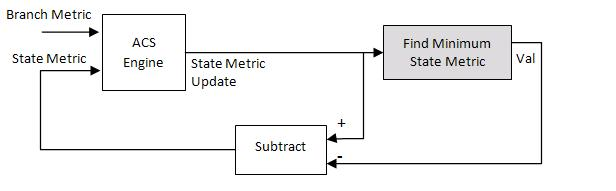# HDL Code Generation from Viterbi Decoder System Object

This example shows how to check, generate and verify HDL code from MATLAB® code that instantiates a Viterbi Decoder System object™.

### MATLAB Design

The MATLAB code used in this example is a Viterbi Decoder used in hard decision convolutional decoding, implemented as a System object. This example also shows a MATLAB test bench that tests the decoder.

```design_name = 'mlhdlc_sysobj_viterbi'; testbench_name = 'mlhdlc_sysobj_viterbi_tb'; ```

Look at the MATLAB design.

```type(design_name); ```
```%%%%%%%%%%%%%%%%%%%%%%%%%%%%%%%%%%%%%%%%%%%%%%%%%%%%%%%%%%%%%%%%%%%%%%%%%% % MATLAB design: Viterbi Decoder % % Key Design pattern covered in this example: % (1) Using comm system toolbox ViterbiDecoder function % (2) The 'step' method can be called only per system object in a design iteration %%%%%%%%%%%%%%%%%%%%%%%%%%%%%%%%%%%%%%%%%%%%%%%%%%%%%%%%%%%%%%%%%%%%%%%%%% % Copyright 2011-2015 The MathWorks, Inc. function decodedBits = mlhdlc_sysobj_viterbi(inputSymbol) persistent hVitDec; if isempty(hVitDec) hVitDec = comm.ViterbiDecoder('InputFormat','Hard', 'OutputDataType', 'Logical'); end decodedBits = step(hVitDec, inputSymbol); ```
```type(testbench_name); ```
```% Viterbi_tb - testbench for Viterbi_dut % Copyright 2011-2015 The MathWorks, Inc. numErrors = 0; % rand stream original_rs = RandStream.getGlobalStream; rs = RandStream.create('mrg32k3a', 'seed', 25); %RandStream.getGlobalStream(rs); rs.reset; % convolutional encoder hConvEnc = comm.ConvolutionalEncoder; % BER hBER = comm.ErrorRate; hBER.ReceiveDelay = 34; reset(hBER); % clear persistent variables in the design between runs of the testbench clear mlhdlc_msysobj_viterbi; for numSymbols = 1:10000 % generate a random bit inputBit = logical(randi([0 1], 1, 1)); % encode it with the Convolutional Encoder - rate 1/2 encodedSymbol = step(hConvEnc, inputBit); % optional - add noise %%%%%%%%%%%%%%%%%%%%%%%%%%%%%%%%%%%%%%%%%%%%%%%%%%%%%%%%% % call Viterbi Decoder DUT to decode the symbol %%%%%%%%%%%%%%%%%%%%%%%%%%%%%%%%%%%%%%%%%%%%%%%%%%%%%%%%% vitdecOut = mlhdlc_sysobj_viterbi(encodedSymbol); ber = step(hBER, inputBit, vitdecOut); end fprintf('%s\n', repmat('%', 1, 38)); fprintf('%%%%%%%%%%%%%% %s %%%%%%%%%%%%%%\n', 'Viterbi Decoder Output'); fprintf('%s\n', repmat('%', 1, 38)); fprintf('Number of bits %d, BER %g\n', numSymbols, ber(1)); fprintf('%s\n', repmat('%', 1, 38)); % EOF ```

### Simulate the Design

Simulate the design with the testbench prior to code generation to make sure there are no runtime errors.

```mlhdlc_sysobj_viterbi_tb ```
```%%%%%%%%%%%%%%%%%%%%%%%%%%%%%%%%%%%%%% %%%%%%% Viterbi Decoder Output %%%%%%% %%%%%%%%%%%%%%%%%%%%%%%%%%%%%%%%%%%%%% Number of bits 10000, BER 0 %%%%%%%%%%%%%%%%%%%%%%%%%%%%%%%%%%%%%% ```

### Hardware Implementation of Viterbi Decoding Algorithm

There are three main components in the Viterbi decoding algorithm. They are the branch metric computation (BMC), add-compare-select (ACS), and traceback decoding. The following diagram illustrates the three units in the Viterbi decoding algorithm.### The Renormalization Method

The Viterbi decoder prevents the overflow of the state metrics in the ACS component by subtracting the minimum value of the state metrics at each time step, as shown in the following figure.Obtaining the minimum value of all the state metric elements in one clock cycle results in a poor clock frequency for the circuit. The performance of the circuit may be improved by adding pipeline registers. However, simply subtracting the minimum value delayed by pipeline registers from the state metrics may still lead to overflow.

The hardware architecture modifies the renormalization method and avoids the state metric overflow in three steps. First, the architecture calculates values for the threshold and step parameters, based on the trellis structure and the number of soft decision bits. Second, the delayed minimum value is compared to the threshold. Last, if the minimum value is greater than or equal to the threshold value, the implementation subtracts the step value from the state metric; otherwise no adjustment is performed. The following figure illustrates the modified renormalization method.### Create a New HDL Coder Project

To create a new project, enter the following command:

```coder -hdlcoder -new mlhdlc_viterbi ```

Next, add the file `mlhdlc_sysobj_viterbi.m` to the project as the MATLAB function and `mlhdlc_sysobj_viterbi_tb.m` as the MATLAB test bench.

For a more complete tutorial on creating and populating MATLAB HDL Coder projects, see Get Started with MATLAB to HDL Workflow.

### Run Fixed-Point Conversion and HDL Code Generation

Launch the Workflow Advisor. In the Workflow Advisor, right-click the Code Generation step and choose the option Run to selected task to run all the steps from the beginning through HDL code generation.

Examine the generated HDL code by clicking the links in the log window.

### Supported System objects

For a list of System objects supported for HDL code generation, see Predefined System Objects Supported for HDL Code Generation.